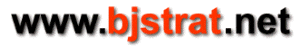## Computing variance for an individual blackjack hand

The variance of an individual blackjack hand that does not involve splitting a pair can be computed from the parameters listed below and their relationships. Below var refers to variance of a given player hand for a given strategy versus a given player up card and vari refers to variance for hand and strategy versus dealer up card of ace given player buys insurance. In order to compare var for a given hand and strategy to vari it should be computed versus up card of ace while vari is computed for the same given hand and strategy (v ace.)

ev is unconditional expected value for a given strategy meaning a non-blackjack loses to dealer blackjack.

## Parameters

pWin = probability of winning the hand
pLose = probability of losing the hand (includes loss to dealer blackjack)
pLose_noDBJ = probability of losing hand where loss is not due to dealer blackjack
pTie = probability of pushing the hand
pDBJ = probability of dealer blackjack
bjOdds = odds paid for a player blackjack
ev = expected value of the hand for a given player strategy versus given dealer up card
evi = expected value of the hand for a given player strategy versus up card of ace given player buys insurance

var = variance (no insurance)
vari = variance (insurance taken)

### Undoubled player blackjack

pWin + pLose + pTie = 1
pLose = 0
pTie = pDBJ
pWin = 1 - pTie
ev(stand v ace) = (1 - pTie)*bjOdds = (1 - pDBJ)*bjOdds
var = pTie*(0 - ev)^2 + pWin*(bjOdds - ev)^2

var = ev^2 + (1 - pTie)*(bjOdds^2 - 2*bjOdds*ev)  can be algebraically derived from above info
evi = bjOdds*(1 - pDBJ) + .5*(3*pDBJ - 1)
vari = pDBJ*(1 - evi)^2 + (1 - pDBJ)*((bjOdds - .5) - evi)^2

special case example:
bjOdds = 1.5 and pDBJ = 1/3
applies to any composition where true
ev = evi = 1
var = .5
vari = 0

### Undoubled hand (non-blackjack)

pWin + pLose + pTie = 1
pWin - pLose = ev
pWin = (1 - pTie + ev)/2
pLose = (1 - pTie - ev)/2
var = pLose*(-1 - ev)^2 + pTie*(0 - ev)^2 + pWin*(1 - ev)^2

var = 1 - pTie - ev^2  can be algebraically derived from above info
evi = ev(up card ace) + .5*(3*pDBJ - 1)
pLose_noDBJ = pLose - pDBJ
vari = pDBJ*(0 - evi)^2 + pLose_noDBJ*(-1.5 - evi)^2 + pTie*(-.5 - evi)^2 + pWin*(.5 - evi)^2

special case examples:
9-7 v ace single deck s17
composition before hand: {4,3,4,4,4,4,4,4,4,16}
composition after hand given up card ace: {3,3,4,4,4,4,3,4,3,16}
ev(hit v ace): -0.67000
pTie, pWin, pLose (hit v ace): 0.03944, 0.14528, 0.81528
var: 0.51166
pDBJ: 16/48 = 1/3
evi = ev(hit v ace) = -0.67000
pLose_noDBJ: 0.48194
vari: 0.68166

T-T v ace single deck s17
composition before hand: {4,3,3,3,3,3,3,3,4,16}
composition after hand given up card ace: {3,3,3,3,3,3,3,3,4,14}
ev(stand v ace): 0.09280
pTie, pWin, pLose (stand v ace): 0.14279, 0.47501, 0.38204
var: 0.84860
pDBJ: 14/42 = 1/3
evi = ev(stand v ace): 0.09280
pLose_noDBJ: 0.04887
vari: 0.25580

### Doubled hand (ENHC)

pWin + pLose + pTie = 1
2*(pWin - pLose) = ev
pWin = (2*(1 - pTie) + ev)/4
pLose = (2*(1 - pTie) - ev)/4
var = pWin*(2 - ev)^2 + pLose*(-2 - ev)^2 + pTie*(0 - ev)^2

var = 4*(1 - pTie) - ev^2  can be algebraically derived from above info
evi = ev(up card ace) + .5*(3*pDBJ - 1)
pLose_noDBJ = pLose - pDBJ
vari = pDBJ*(0 - evi)^2 + pLose_noDBJ*(-2.5 - evi)^2 + pTie*(-.5 - evi)^2 + pWin*(1.5 - evi)^2

special case example:
6-5 v ace single deck s17
composition before hand: {4,3,4,4,4,4,4,4,4,16}
composition after hand given up card ace: {3,3,4,4,3,3,4,4,4,16}
ev(dbl v ace): -0.48724
pTie, pLose, pWin (dbl v ace): 0.05024, 0.51336, 0.43640
var: 3.56161
pDBJ: 16/48 = 1/3
evi = ev(dbl v ace): -0.48724
pLose_noDBJ: 0.18002
vari: 2.53185

### Doubled hand (Full peek)

pWin + pLose + pTie = 1
2*(pWin - pLose) + pDBJ = ev
pWin = (2*(1 - pTie) + ev)/4
pLose = (2*(1 - pTie) - ev)/4
pLose_noDBJ = pLose - pDBJ
var = pWin*(2 - ev)^2 + pLose_noDBJ*(-2 - ev)^2 + pTie*(0 - ev)^2 + pDBJ*(-1 - ev)^2

var = 4*(1 - pTie) - 3*pDBJ - ev^2  can be algebraically derived from above info
evi = ev(up card ace) + .5*(3*pDBJ - 1)
vari = pDBJ*(0 - evi)^2 + pLose_noDBJ*(-2.5 - evi)^2 + pTie*(-.5 - evi)^2 + pWin*(1.5 - evi)^2

special case example:
6-5 v ace single deck s17
composition before hand: {4,3,4,4,4,4,4,4,4,16}
composition after hand given up card ace: {3,3,4,4,3,3,4,4,4,16}
ev(dbl v ace): -0.15391
pTie, pLose, pWin (dbl v ace): 0.05024, 0.51336, 0.43640
var: 2.77533
pDBJ: 16/48 = 1/3
evi = ev(dbl v ace): -0.15391
pLose_noDBJ: 0.18002
vari: 2.19851

## Computing variance for pair split

The variance of a pair split can also be computed but is more complicated. If number of allowed splits is 1 then the range of units won/lost is -2 to +2 if there is no doubling after splitting and the range is -4 to +4 if doubling after splitting is allowed. Each additional allowed split increases this range. To compute variance the probability of each possible units won/lost needs to be computed. The problem with this is that as number of decks and number of allowed splits increases the number of required iterations grows exponentially. For each necessary iteration dealer probabilites need to be calculated. I am able to get values for single deck, 1 allowed split which takes a while and 2 decks, 1 allowed split which takes even longer.